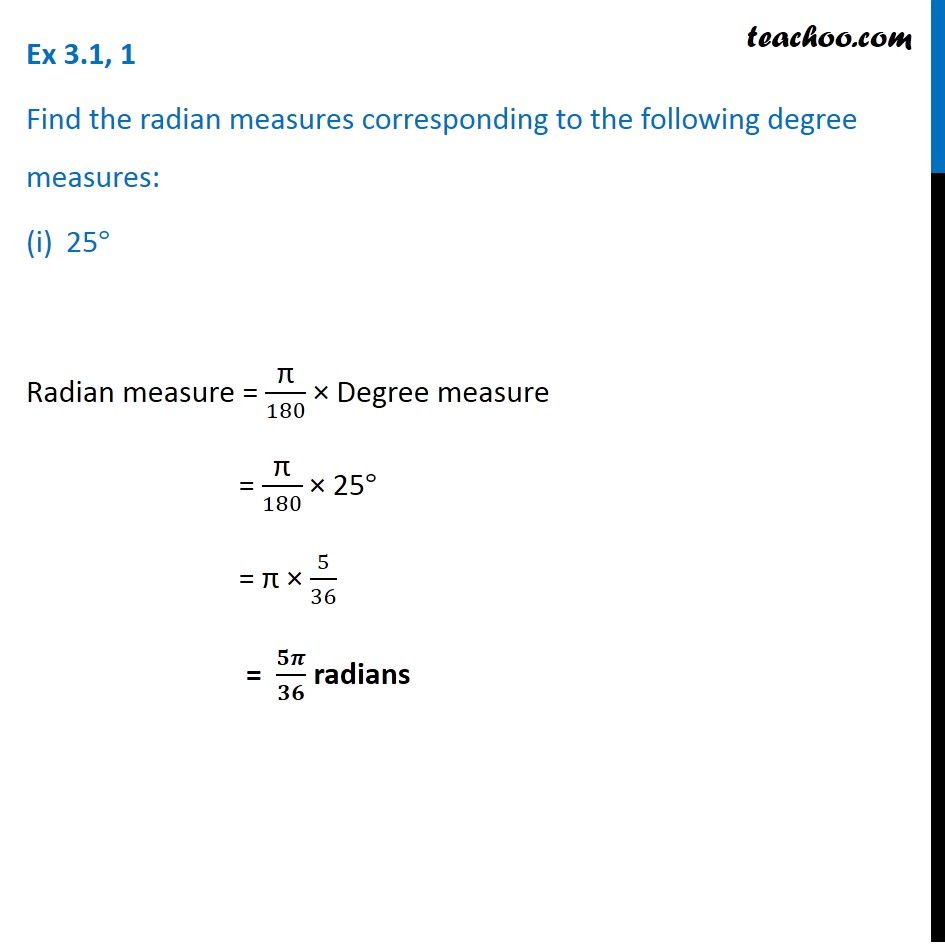1. Chapter 3 Class 11 Trigonometric Functions (Term 2)
2. Concept wise

Transcript

Ex 3.1, 1 Find the radian measures corresponding to the following degree measures: 25° Radian measure = "π" /180 × Degree measure = "π" /180 × 25° = "π" × 5/36 = 𝟓𝝅/𝟑𝟔 radians